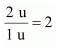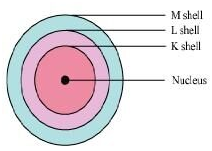• +91 9971497814
• info@interviewmaterial.com

# Chapter 4 Structure Of The Atom Questions Answers

Question - 1 : -
What are canal rays?

Canal rays are positively charged radiations. These rays consist of positively charged particles known as protons. They were discovered by Goldstein in 1886.

Question - 2 : -
If an atom contains one electron and one proton, will it carry any charge or not?

An electron is a negatively charged particle, whereas a proton is a positively charged particle. The magnitude of their charges is equal. Therefore, an atom containing one electron and one proton will not carry any charge. Thus, it will be a neutral atom.

Question - 3 : -
On the basis of Thomson’s model of an atom, explain how the atom is neutral as a whole.

According to Thomson’s model of the atom, an atom consists of both negatively and positively charged particles. The negatively charged particles are embedded in the positively charged sphere. These negative and positive charges are equal in magnitude. Thus, by counterbalancing each other’s effect, they make an atom neutral.

Question - 4 : -
Name the three sub-atomic particles of an atom.

The three sub-atomic particles of an atom are:

(i) Protons

(ii) Electrons, and

(iii) Neutrons

Question - 5 : -
On the basis of Rutherford’s model of an atom, which subatomic particle is present in the nucleus of an atom?

On the basis of Rutherford’s model of an atom, protons (positively-charged particles) are present in the nucleus of an atom.

Question - 6 : -
Helium atom has an atomic mass of 4 u and two protons in its nucleus. How many neutrons does it have?

Answer - 6 : - A helium atom has two neutrons. The mass of an atom is the sum of the masses of protons and neutrons present in its nucleus. Since helium atom has two protons, mass contributed by the two protons is (2 × 1) u = 2 u. Then, the remaining mass (4 − 2) u = 2 u is contributed byneutrons

Question - 7 : -
Draw a sketch of Bohr’s model of an atom with three shells.

Answer - 7 : -Bohr’s model of an atom with three shells

Question - 8 : -
What do you think would be the observation if the α-particle scattering experiment is carried out using a foil of a metal other than gold?

If the α-scattering experiment is carried out using a foil of a metal rather than gold, there would be no change in the observation. In the α-scattering experiment, a gold foil was taken because gold is malleable and a thin foil of gold can be easily made. It is difficult to make such foils from other metals.

Question - 9 : -
Write the distribution of electrons in carbon and sodium atoms?

The total number of electrons in a carbon atom is 6. The distribution of electrons in carbon atom is given by:

First orbit or K-shell = 2 electrons
Second orbit or L-shell = 4 electrons

Or, we can write the distribution of electrons in a carbon atom as 2, 4.

The total number of electrons in a sodium atom is 11. The distribution of electrons in sodium atom is given by:
First orbit or K-shell = 2 electrons
Second orbit or L-shell = 8 electrons
Third orbit or M-shell = 1 electron

Or, we can write distribution of electrons in a sodium atom as 2, 8, 1.

Question - 10 : -
If K and L shells of an atom are full, then what would be the total number of electrons in the atom?

The maximum number of electrons that can occupy K and L-shells of an atom are 2 and 8 respectively. Therefore, if K and L-shells of an atom are full, then the total number of electrons in the atom would be (2 + 8) = 10 electrons.

Todays Deals### Chapter 4 Structure Of The Atom Contributorskrishan

Name:
Email:

# Latest News# 9000 interview questions in different categories